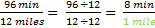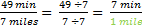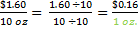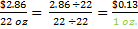# Unit Rates

A unit rate is a comparison where the second value is 1. This could be miles driven per hour (one hour), the cost of one apple, the words you can type in a minute. Unit rates are helpful when trying to compare two quantities.

Example: Billy drove 300 miles in 5 hours. Carlos drove 440 miles in 8 hours. Who drove faster?

To figure this out, we first find the unit rates for each driver.

Billy:Here we can see that Billy was driving at an average speed of 60 miles per hour.

Carlos:So Carlos is driving at an average speed of 55 miles per hour. Now that we have the unit rates, we can see that Billy was driving faster.

Example: Hannah ran 12 miles in 96 minutes. Sunil ran 7 miles in 49 minutes. Who was running faster?

Again, we will calculate the unit rates first.

Hannah:This unit rate tells us that Hannah can run a mile in about 8 minutes.

Sunil:We can see that Sunil is running each mile in only 7 minutes. In this case, the lower number is faster. We can see that it takes Sunil less time to run a mile and the lower time is the faster person.

Example: A 10 oz. bottle of juice is \$1.60 and a 22 oz. bottle of juice is \$2.86. Which bottle is the better buy?

Bottle #1:The unit rate shows that the cost per ounce is \$0.16.

Bottle #2:The unit rate for the second bottle is \$0.13 per ounce.

Comparing the two unit rates, we can see that the second bottle is cheaper per ounce and is therefore, the better buy!

In order to compare to rates, it is important to get a unit rate so that you can compare similar items.